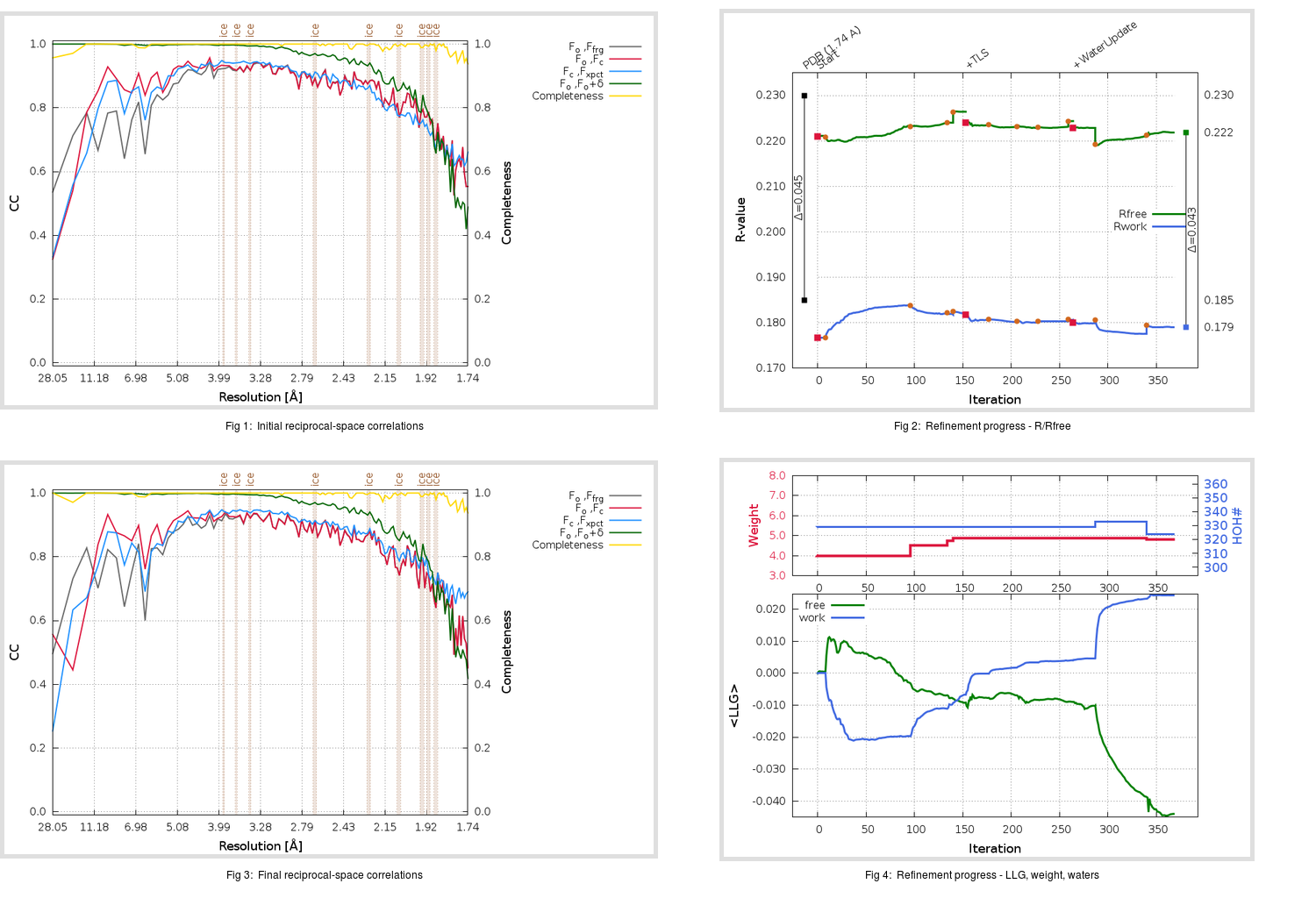Content:

```    Diffraction limits & principal axes of ellipsoid fitted to diffraction cut-off surface:
1.941         0.9987   0.0000  -0.0515       0.994 a* - 0.108 c*
1.664         0.0000   1.0000   0.0000       b*
1.718         0.0515   0.0000   0.9987       0.134 a* + 0.991 c*
```

## Deposited

` `
 Date deposited Date data collection Resolution R, Rfree 20200315 20200303 1.74 0.1830 0.2300

Molprobity (CCP4 7.0 version) summary:

```Ramachandran outliers =   0.33 %
favored =  97.35 %
Rotamer outliers      =   0.38 %
C-beta deviations     =     0
Clashscore            =   4.22
RMS(bonds)            =   0.0131
RMS(angles)           =   1.80
MolProbity score      =   1.33
Resolution            =   1.74
R-work                =   0.1830
R-free                =   0.2300
```

```Number of waters      =   329

<B> (all atoms) =   24.54 ( sd =    8.99 ) for       2730 non-hydrogen atoms
<B>   (protein) =   23.16 ( sd =    8.12 ) for       2370 non-hydrogen atoms
<B>     (water) =   33.86 ( sd =    9.28 ) for        329 non-hydrogen atoms
<B>    (others) =   31.65 ( sd =   11.22 ) for         31 non-hydrogen atoms

B min/max       (all non-hydrogen atoms) =   11.83 /   67.30
B min/max   (protein non-hydrogen atoms) =   12.74 /   67.17
B min/max     (water non-hydrogen atoms) =   11.83 /   67.30
B min/max     (other non-hydrogen atoms) =   22.87 /   43.12
```

## BUSTER (re-)refinement

` `

Molprobity (CCP4 7.0 version) summary:

```Ramachandran outliers =   0.33 %
favored =  98.34 %
Rotamer outliers      =   0.76 %
C-beta deviations     =     0
Clashscore            =   2.32
RMS(bonds)            =   0.0114
RMS(angles)           =   1.56
MolProbity score      =   1.01
Resolution            =   1.74
R-work                =   0.1791
R-free                =   0.2219
```

```Number of waters      =   324

<B> (all atoms) =   26.07 ( sd =    8.67 ) for       2725 non-hydrogen atoms
<B>   (protein) =   24.50 ( sd =    7.26 ) for       2370 non-hydrogen atoms
<B>     (water) =   36.94 ( sd =    9.98 ) for        324 non-hydrogen atoms
<B>    (others) =   32.66 ( sd =   12.27 ) for         31 non-hydrogen atoms

B min/max       (all non-hydrogen atoms) =   13.83 /   70.68
B min/max   (protein non-hydrogen atoms) =   14.57 /   67.89
B min/max     (water non-hydrogen atoms) =   13.83 /   70.68
B min/max     (other non-hydrogen atoms) =   25.76 /   44.04
```

Refinement progression:Results:

` `
 File Remark 5REK_aB_refine.01_03_refine.pdb.gz exact refinement commands are in header 5REK_aB_refine.01_03_refine.mtz.gz including original deposited data and several re-refinement map coefficients 5REK_aB_refine.01_03_BUSTER_model.cif.gz including any non-standard compound restraints 5REK_aB_refine.01_03_BUSTER_refln.cif.gz